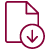Click on this iconto view the PDF for the project.

Click on this iconto play the video for the project on YouTube.

## Track 8A - Poster Presentations

### COGNITIVE SCIENCE AND THE EDUCATION MATHEMATICS

Presenter(s): Amy Sullivan

Addition is regarded as a fundamental part of mathematical practice. Yet, we do not understand how students construct their understanding of this operation. This project will look at three methods of addition and then discuss the underlying logical systems that give rise to these methods. We will then consider the cognitive neuroscience and the human reasoning in mathematics behind one of these methods. Finally, we will consider some teaching methods and strategies that align with a supposed model of student learning.

### EXPLORING SURVIVAL ANALYSIS ASSUMING STOCHASTIC ORDER

Presenter(s): Kendell Visser

The Kaplan Meier Estimator is the most well-known survival curve estimator in the branch of biostatistics. However, when the Kaplan Meier estimator is found for two datasets assuming a certain condition known as stochastic order, the estimator can violate the assumption, which presents some issues of bias and mean squared error. For example, we would expect a Degree 1 Carcinoma Cancer patient to have higher survival probabilities and an increased likelihood of living longer than a Degree 2 Carcinoma Cancer patient because of their slightly healthier condition. However, the survival functions we derived from Carcinoma Cancer datasets (survival package in R studio, Stanford University, 1977) cross over each other with respect to survival probability and time, which is a violation of stochastic ordering. In this project, we will consider an estimator proposed by Rojo (2004) to force stochastic ordering as we evaluate the Carcinoma Cancer data in the two-sample case as well as look into a theoretical three-sample case using exponential data created using R studio.

### INCORPORATING MOVEMENT INTO MATHEMATICS INSTRUCTION

Presenter(s): Brooke Arrieta

In this project we investigate the benefits of incorporating movement into the mathematics classroom. We also look at methods for implementing movement into a secondary mathematics lesson with a focus on pedagogical techniques that include active and tactile learning.

### MODELING HUNTING ENERGY EXPENDITURE

Presenter(s): Lew Griffith